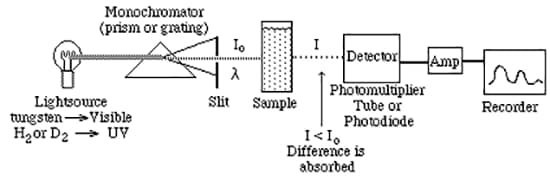# What goes on under a spectrophotometer’s cover?As seen in the figure above, a spectrophotometer measures the light that passes through the sample, to then strike the detector, where it is measured. Let’s call the amount of light at wavelength λ incident on the sample cuvette Io and the amount of light exiting the sample to hit the detector I. If we now calculate the percent of the ratio these two values (I/Io)*100 we get a percent transmission (%T) value. If the sample transmits all the light at a given wavelength then %T = 100; however, if the sample absorbs light then we will have the case where I < Io and %T will be a number less than 100. If the beam is totally blocked or absorbed by the sample, then %T = 0.

One more important item. When a spectrophotometer is turned on it is literally as “dumb as a stump”. In other words, the instrument has not been calibrated for the values of 100 %T or 0 %T. This 100 %T calibration is acquired by a process called background correction for a spectrum. it is also sometimes called auto-zero for a single wavelength measurement. A background correction is performed by removing the sample from the instrument and measuring the amount of light that strikes the detector in the scan range of all wavelengths in the spectrum. These 100 %T values (called a background scan) are then stored in memory for use in calculating accurate sample %T values for a sample.

This brings us to the main disadvantage of single beam instruments, a phenomenon known as drift. There are numerous components in an instrument that are not stable over time (usually due to electrical fluctuations or heat buildup). The lamps and detector are subject to variations in electrical output while electronic resistance values on circuit boards can change due to heat buildup in the instrument. The net effect is that %T values can change over time after the background correction is performed. This means that frequent and timely background corrections must be performed in conjunction with sample measurements. A solution to the “time drift problem” is the double beam instrument.

Back to Index